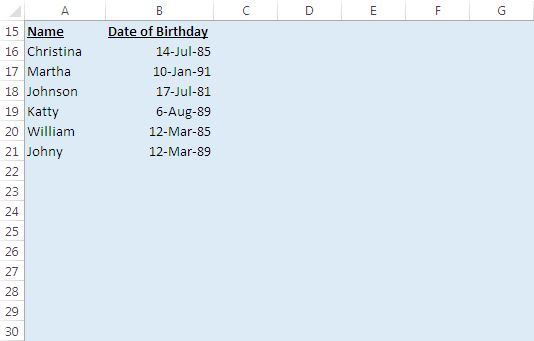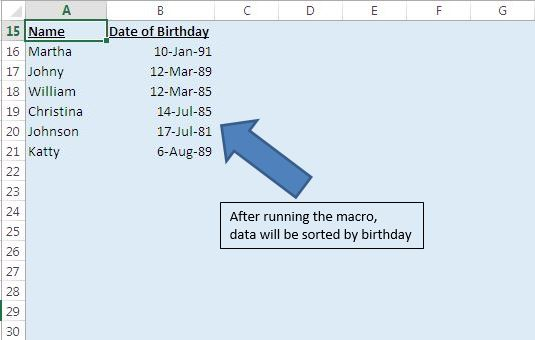# Sort the data by birthday using VBA in Microsoft Excel

In this article, we will create a macro for sorting data in the sheet by birthday and we will not consider year while sorting the data.

Raw data consists of two columns, one contains the name and second column contains the birth date.Logic explanation

We have created a macro, “sorting_names_by_birthday” which will sort the data.

In this macro, firstly we insert formulas in the column C where we find the difference between the birth date and first date of their birth year. Then we sort the data by Name for sorting data in alphabetical order so that if two candidates have same birth date then their names should appear in alphabetical order. Then we sort the data by calculating difference in ascending order to sort data by birthday. After sorting the data, for deleting the formulas from the column C, delete the entire column C.

For running the macro, Press Alt +F8 or Go to Developer tab > click on Macro.```
Option Explicit

Sub sorting_names_by_birthday()

'Disabling screen update
Application.ScreenUpdating = False

Dim Last_Row As Long

'Finding the last row
Last_Row = ActiveCell.SpecialCells(xlCellTypeLastCell).Row

Range("C16").Select

'Getting the days of the year
'Subtracting first date of the year from date of birthday
ActiveCell.FormulaR1C1 = "=RC[-1]-DATE(YEAR(RC[-1]),1,1)"

'Dragging the formula
Range("C16:C" & Last_Row).Select
Selection.FillDown

'Sorting the data first by column A then by column C
Range("A15").CurrentRegion.Sort _
key1:=Range("C15"), order1:=xlAscending, _
key2:=Range("A15"), order2:=xlAscending, _

'Deleting the column C
Columns("C").Delete

Range("A15").Select

End Sub

```